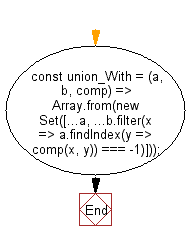# JavaScript: Find every element that exists in any of the two given arrays once

## JavaScript fundamental (ES6 Syntax): Exercise-27 with Solution

Write a JavaScript program to find every element that exists in any of the two given arrays once, using a provided comparator function.

• Create a new Set() with all values of a and values in b for which the comparator finds no matches in a, using Array.prototype.findIndex().

Sample Solution:

JavaScript Code:

``````//#Source https://bit.ly/2neWfJ2
const union_With = (a, b, comp) =>
Array.from(new Set([...a, ...b.filter(x => a.findIndex(y => comp(x, y)) === -1)]));

console.log(union_With([1, 1.2, 1.5, 3, 0], [1.9, 3, 0, 3.9], (a, b) => Math.round(a) === Math.round(b)));
console.log(union_With([1, 2, 3, 4], [1, 2, 3, 4, 5], (a, b) => Math.round(a) === Math.round(b)));
```
```

Sample Output:

```[1,1.2,1.5,3,0,3.9]
[1,2,3,4,5]
```

Flowchart:Live Demo:

See the Pen javascript-basic-exercise-1-27 by w3resource (@w3resource) on CodePen.

Improve this sample solution and post your code through Disqus

What is the difficulty level of this exercise?

Test your Programming skills with w3resource's quiz.

﻿

## JavaScript: Tips of the Day

Chunks an array into n smaller arrays

Example:

```const tips_chunkIntoN = (arr, n) => {
const size = Math.ceil(arr.length / n);
return Array.from({ length: n }, (v, i) =>
arr.slice(i * size, i * size + size)
);
}
console.log(tips_chunkIntoN([1, 2, 3, 4, 5, 6, 7,8], 4));
```

Output:

```[[1,2],[3,4],[5,6],[7,8]]
```